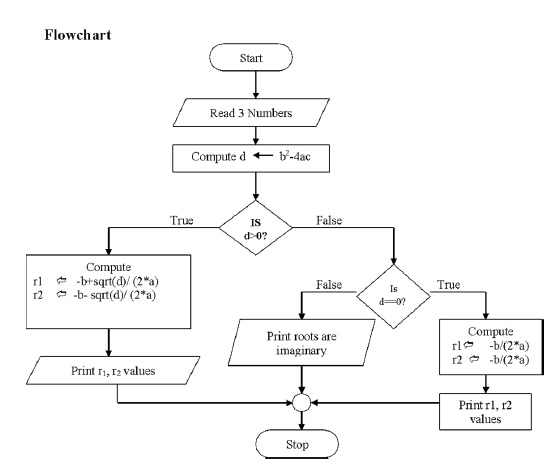# How to write a C program to find the roots of a quadratic equation?

## Problem

Applying the software development method to solve any problem in C Language

## Solution

• Find roots of a quadratic equation, ax2+bx+c.
• There will be 2 roots for given quadratic equation.

## Analysis

Input − a,b,c values

Output − r1, r2 values

## Procedure

$r_{1}=\frac{-b+\sqrt{b^2-4ac}}{2a}$

$r_{2}=\frac{-b-\sqrt{b^2-4ac}}{2a}$

## Design (Algorithm)

• Start
• Read a, b, c values
• Compute d = b2 4ac
• if d > 0 then
• r1 = b+ sqrt (d)/(2*a)
• r2 = b sqrt(d)/(2*a)
• Otherwise if d = 0 then
• compute r1 = -b/2a, r2=-b/2a
• print r1,r2 values
• Otherwise if d < 0 then print roots are imaginary
• Stop## Implementation Code

# include<stdio.h>
# include<conio.h>
# include<math.h>
main (){
float a,b,c,r1,r2,d;
printf (“enter the values of a b c”);
scanf (“ %f %f %f”, &a, &b, &c);
d= b*b – 4*a*c;
if (d>0){
r1 = -b+sqrt (d) / (2*a);
r2 = -b-sqrt (d) / (2*a);
printf (“The real roots = %f %f”, r1, r2);
}
else if (d= =0){
r1 = -b/(2*a);
r2 = -b/(2*a);
printf (“roots are equal =%f %f”, r1, r2);
}
else
printf(“Roots are imaginary”);
getch ();
}

## Testing

Case 1: enter the values of a b c: 1 4 3
r1 = -1
r2 = -3
Case 2: enter the values of a b c: 1 2 1
r1 = -1
r2 = -1
Case 3: enter the values of a b c: 1 1 4
Roots are imaginary